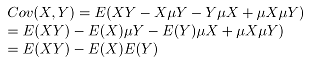# 协方差与相关系数

﻿﻿

### 协方差

 160cm 170cm 180cm 60kg 0.2 0.05 0.05 70kg 0.05 0.3 0.05 80kg 0.05 0.05 0.2### 定义

Cov(X,Y)=E[(Xμ X )(Yμ Y )]

 160cm 170cm 180cm 60kg 0.2 0.05 0.05 70kg 0.05 0.3 0.05 80kg 0.05 0.05 0.2

 160cm 170cm 180cm 60kg 100 0 -100 70kg 0 0 0 80kg -100 0 100

Cov(X,Y) =0.2×100+0.2×100+0.05×(100)+0.05×(100)=30(注意，Cov(X,Y)=0 并不意味着X和Y独立)

### 相关系数

ρ=Cov(X,Y)Var(X)Var(Y) − − − − − − − − − − − −

Var(X)=0.3×(6070) 2 +0.3×(8070) 2 =60

Var(Y)=0.3×(180170) 2 +0.3×(160170) 2 =60

ρ=30/60=0.5